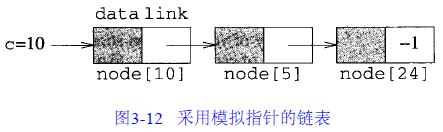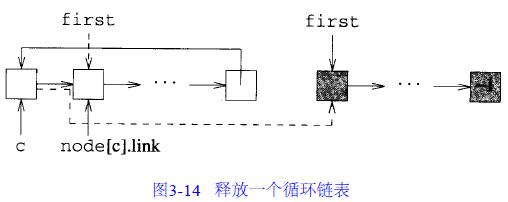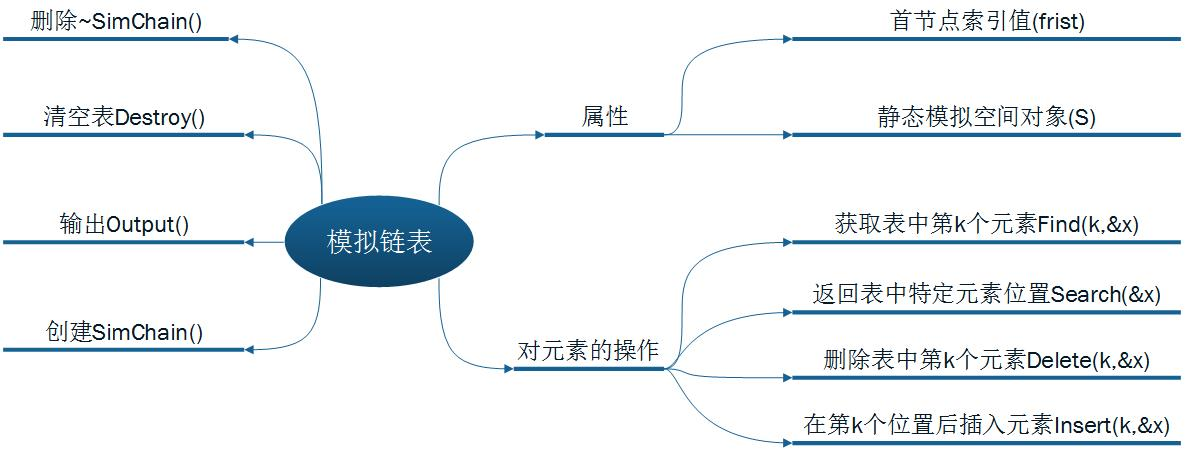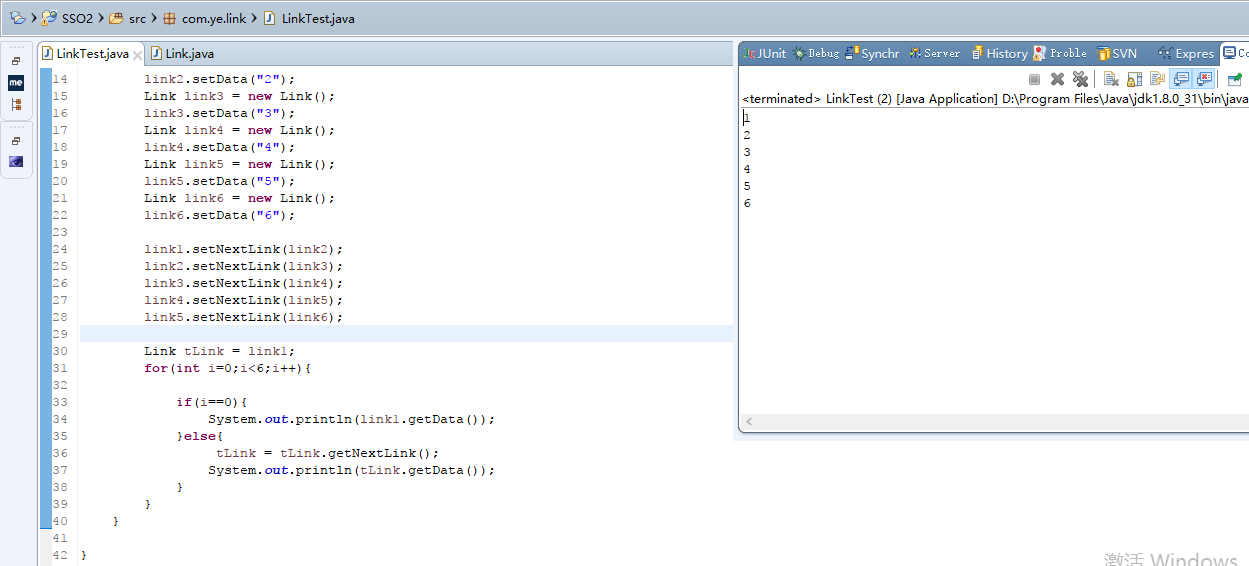• 模拟指针

千次阅读 2017-07-07 09:26:45
有时候采用一个节点数组以及对该数组进行索引的模拟指针，可以使设计更方便、更高效。

折腾了相当长一段时间，今天终于把代码整理好了，小编是个自学的beginner，可以看到有些文字还只是摘抄的，好不容易才弄出一篇有自己想法的东西。我目前的学习策略是，先把目标算法的相关代码实现了，然后整体阅读代码，在代码中加上自己的看法。代码书上是有的，但是结构分散，有些细节的东西并没有说明清楚，这对初学者相当考验。关于模拟指针，在网上搜索一下，不知道是自己搜索方式不好还是怎么，得到的中文信息较少，基本上也是把书上的东西贴上。我没有搜索英文方面的文章。

由于小编水平有限，发表的看法可能会有误，有不好的地方请指教。

有时候采用一个节点数组以及对该数组进行索引的模拟指针，可以使设计更方便、更高效。模拟指针的类定义及其操作
Allocate从存储池中取出节点，每次取出一个。Deallocate则将节点放入存储池中，每次放入一个。存储池为可用空间表。
这是SimSpace类，模拟可用空间表

#pragma once
#include<iostream>
//#include"SimulChain.h"
using namespace std;

template <class T>
class SimSpace
{
friend class SimChain<T>;
public:
SimSpace(int MaxSpaceSize = 100);
~SimSpace() { delete[] node; }
int Allocate(); //分配一个节点
void Deallocate(int& i); //释放节点i
template<class T>
class SimNode   //刚开始并不知道把SimNode类作为SimSpace类的嵌套类，
//这两个类彼此有利用，把谁放到前面都不合适。
{
friend class SimSpace<T>;
friend class SimChain<T>;
private:
T data;
};

private:
int NumberOfNodes, first;
SimNode<T> *node;//节点数组
};

template<class T>
SimSpace<T>::SimSpace(int MaxSpaceSize)
{
// 构造函数
NumberOfNodes = MaxSpaceSize;
node = new SimNode<T>[NumberOfNodes];
// 初始化可用空间表
// 创建一个节点链表
for (int i = 0; i < NumberOfNodes - 1; i++)
// 链表的最后一个节点
// 链表的第一个节点
first = 0;
};

template<class T>
int SimSpace<T>::Allocate()
{
//这个操作相当于删除可用空间表中的第一个节点，拿出来用了
// 分配一个自由节点
if (first == -1) throw NoMem();
int i = first; //分配第一个节点
return i;
};

template<class T>
void SimSpace<T>::Deallocate(int& i)
{
//这个函数相当于在可用空间表中添加一个节点，存进去了
// 释放节点i .
// 使i 成为可用空间表的第一个节点
first = i;
//i = -1;  //这一步是为什么？这个注释与否对目前的测试代码的结果表面上没有影响。
};

//使用两个空间表的部分函数版本，需要把first1和first2设置位SimSpace的私有成员
//template<class T>
//SimSpace<T>::SimSpace(int MaxSpaceSize)
//{
//  // 使用两个可用空间表的构造函数
//  NumberOfNodes = MaxSpaceSize;
//  node = new SimNode<T>[NumberOfNodes];
//  // 初始化可用空间表
//  firstl = 0;
//  first2 = -1;     //模拟指针和C++指针的区别
//};
//
//template<class T>
//int SimSpace<T>::Allocate()
//{
//  // 分配一个自由节点
//  if (first2 == -1) {// 第2个表为空
//      if (firstl == NumberOfNodes) throw NoMem();
//      return firstl++;
//      }
//  // 分配链表中的第一个节点
//  int i = first2;
//  return i;
//}

————————
采用可用空间表模式分解一个链表将比采用C + +指针更高效。例如，如果一个单向链表的首部和尾部分别为f 和e，可以采用如下语句来释放链表中的所有节点：

node[e].link = first; first = f;  //既然所有节点都在链表中，那么可用空间表中的自由节点为零，所以first等于-1？

————————
如果c 是一个循环链表，则采用如下程序释放表中所有节点

template<class T>
void SimSpace<T>::DeallocateCircular(int& c)
{
// 释放一个循环链表c
if (c != -1) {
first = next;
c = -1;
}
};这是SimChain类。

#pragma once
#include<iostream>
//#include"SimulSpace.h"  //这里包不包含都对下面SimChain类使用SimSpace类无帮助
//一直都是不会划红线但是编译会报奇怪的错误，
using namespace std;

template<class T> class SimSpace;   //在这里添加了这句，编译就没有报出那个错误了
//但是类模板声明和定义不是应该放在一起的吗？

template<class T>
class SimChain
{
public:
SimChain() { first = -1; }
~SimChain() { Destroy(); }
void Destroy(); // 使表为空
int Length() const;
bool Find(int k, T& x) const;
int Search(const T& x) const;
SimChain<T>& Delete(int k, T& x);
SimChain<T>& Insert(int k, const T& x);
void Output(ostream& out) const;
private:
int first; // 第一个节点的索引
static SimSpace<T> S;
};

template<class T>
void SimChain<T>::Destroy()
{
// 释放链表节点
int next;
while (first != -1)
{
S.Deallocate(first);
first = next;
}
}

template<class T>
int SimChain<T>::Length() const
{
// 返回链表的长度。我们可以算出链表的长度，
//但是无法计算出可用空间的长度？计算可用空间的长度也没有意义？
int current = first, // 链节点的当前位置
len = 0; //元素计数
while (current != -1)
{
len++;
}
return len;
}

template<class T>
bool SimChain<T>::Find(int k, T& x) const
{
// 取第k个元素至x
// 如果不存在第k个元素，函数返回false，否则返回true
if (k < 1) return false;
int current = first, // 链节点的当前位置
index = 1; //当前节点的索引
// 移动current至第k个节点
while (index < k && current != -1)
{
index++;
}
// 验证是否到达了第k个节点
if (current != -1)
{
x = S.node[current].data;
return true;
}
return false; // 不存在第k个元素
}

template<class T>
SimChain<T>& SimChain<T>::Delete(int k, T& x)
{
// 把第k个元素取至x，然后删除第k个元素
// 如果不存在第k个元素，则引发异常OutOfBounds
if (k < 1 || first == -1)
throw OutOfBounds(); // 不存在第k个元素
// p最终将指向第k个节点
int p = first;
// 将p移动至第k个节点，并从链表中删除该节点
if (k == 1) // p已经指向第k个节点
//这里表明k与数值node中的下标i是没有必然联系的。
//k==1定位到SimChain链表中的第一个节点，first指向这个链表
//中的第一个节点，但是node[first]可以是数组中的任何位置的元素
else
{
// 使用q指向第k - 1个元素
int q = first;
for (int index = 1; index < k - 1 && q != -1; index++)
// 验证第k个元素的存在性
if (q == -1 || S.node[q].link == -1)
throw OutOfBounds(); // 不存在第k个元素
// 使p指向第k个元素
// 从链表中删除第k个元素
//数组中的下标，传给Deallocate函数的也是这个下标,
//从这里可以得到一条经验：传给SimSpace表相关操作
//的参数应该都是node数组的下标。而k这里是SimChain链表
//索引。
}
// 保存第k个元素并释放节点p
x = S.node[p].data;
S.Deallocate(p);  //释放这个已经删除的节点的目的就是为了让这个节点回归到
//可用空间表中。否则，它就处于“游离”状态，下次就没法使用，
//相当于“内存泄漏”了。
return *this;
}

template<class T>
SimChain<T>& SimChain<T>::Insert(int k, const T& x)
{
// 在第k个元素之后插入x
// 如果不存在第k个元素，则引发异常OutOfBounds
// 如果没有足够的空间，则传递NoMem异常
if (k < 0)
throw OutOfBounds();
// 定义一个指针p，p最终将指向第k个节点
int p = first;
// 将p移向第k个节点
for (int index = 1; index < k && p != -1; index++)
// 验证第k个节点的存在性
if (k > 0 && p == -1)
throw OutOfBounds();
// 为插入操作分配一个新节点
int y = S.Allocate();
S.node[y].data = x;
// 向链表中插入新节点
// 首先检查新节点是否要插到链表的首部
if (k)
{
//在p之后插入
}
else
{
// 作为链表首节点
S.node[y].link = first; first = y;
}
return *this;
}

template<class T>
int SimChain<T>::Search(const T& x) const
{
// 寻找x，如果发现x，则返回x的地址
// 如果x不在链表中，则返回0
int current = first,  //链节点的当前位置
index = 1; // current的索引
while (current != -1 && S.node[current].data != x)
{
index++;
}
if (current != -1)
return index;
return 0;
}

template<class T>
void SimChain<T>::Output(ostream& out) const
{
// 将链表元素送至输出流
int current;
for (current = first; current != -1; current = S.node[current].link)
}

// 重载<<
template <class T>
ostream& operator<<(ostream& out, const SimChain<T>& x)
{
x.Output(out);
return out;
}

异常处理

#pragma once
#include <iostream>
using namespace std;
// 内存不足
class NoMem
{
public:
NoMem() {}
};
// 使new引发NoMem异常而不是xalloc异常
void my_new_handler()
{
throw NoMem();  //什么意思
}
new_handler Old_Handler_ = set_new_handler(my_new_handler);

class OutOfBounds
{
public:
OutOfBounds() {}
};

主函数

//头文件包含的顺序很重要，在两个头文件中分别不包含彼此但有相互引用
//的情况下，Space.h对Chain.h的依赖比反之更强。在目前这种包含关系下，编译
//产生的错误跟之前的错误一样，即在两个头文件都彼此包含的情况下编译产生的错误。
//这也就是说，在头文件和源文件都包含了相关文件的情况下，编译器选择了按源文件中的包含顺序
//来执行？那我现在把源文件中的相关头文件注释掉，然后在两个自编头文件中包含彼此，会怎么样？
//原文件一注释掉就划红线，所以不能这样。现在搞清楚了一个关系，同一个项目中的头文件，只需要
//在源文件中包含就行了，但这要注意顺序！

//不得不注意，SimChain类和SimSpace类中都有一个first变量，应该区分清楚，
//SimChaim.first初始化为-1，SimSpace初始化为0。
//这是很自然的，从各个类的结构中看，实例化后，可用空间充满自由节点，
//它的first理应指向第一个节点（first=0）；而SimChain链表还没有节点，所以它的first=-1。

#include <iostream>
#include "SimulChain.h"
#include "SimulSpace.h"
#include "Xcept.h"

using namespace std;

SimSpace<int> SimChain<int>::S; //实例化可用空间S（调用SimSpace类构造函数），
//这时存在一个足够大的数组，存放很多节点,

void main(void)
{
int x;
SimChain<int> c;
cout << "Chain length is" << c.Length() << endl;
c.Insert(0, 2).Insert(1, 6);
cout << "Chain length is" << c.Length() << endl;
c.Find(1, x);
cout << "First element is" << x << endl;
c.Delete(1, x);
cout << "Deleted" << x << endl;
cout << "New length is" << c.Length() << endl;
cout << "Position of 2 is" << c.Search(2) << endl; //此处返回了0，表示不已经在链表中
cout << "Position of 6 is" << c.Search(6) << endl;
c.Insert(0, 9).Insert(1, 8).Insert(2, 7);
cout << "Current chain is" << c << endl;
cout << "Its length is" << c.Length() << endl;
cout << "Position of 2 is" << c.Search(2) << endl;
cout << "Position of 6 is" << c.Search(6) << endl;
}

以上内容部分整理自网络电子资料，仅供学习交流用，勿作商业用途。转载请注明来源。

展开全文算法 模拟指针
• 1、相关概念1.1、模拟指针的定义模拟指针可以理解为：在一个模拟空间中，存在一个指针数组，数组中的每个元素为指针类型，并且每个指针具有数据域和链接域（指向下一个数组中的指针）。1.2、模拟指针描述单链表、...

1、相关概念

1.1、模拟指针的定义

模拟指针可以理解为：在一个模拟空间中，存在一个指针数组，数组中的每个元素为指针类型，并且每个指针具有数据域和链接域（指向下一个数组中的指针）。

1.2、模拟指针描述单链表、间接寻址描述单链表及普通单链表之间的区别：

1. 普通单链表中，各个节点没有索引值；
2. 间接寻址描述单链表时，各个节点间的索引值满足一定的数学关系；
3. 模拟指针描述单链表时，各个节点的索引值不用满足一定的数学关系，但每个节点的索引值唯一。

2、模拟指针的类定义及实现

利用C++语言，实现模拟指针的类定义，包括模拟空间类SimSpace和模拟节点类SimNode。初始时，模拟空间中存放了所有的节点（MaxSize个）。为了在模拟空间中实现模拟指针，那么，就必须在需要新节点的时候申请一个指向该节点的指针（从模拟空间中取出一个节点）；同理，在不需要此节点的时候释放此节点的指针（向模拟空间中放入一个节点）。相当于C++函数new和delete。在程序中，利用Allocate()函数和Deallocate()函数实现。为了简单起便，上述操作在模拟空间的前部进行。

2.1 模拟指针的类定义

template <class T> class SimSpace;
template <class T> class SimChain;
template <class T>
class SimNode
{
friend SimSpace<T>;        //友类模拟空间类
friend SimChain<T>;        //友类模拟链表类

private:
T data;                    //数据
};
template <class T> class SimChain;
template <class T>
class SimSpace
{
friend SimChain<T>;
public:
SimSpace(int MaxSize=100);
~SimSpace();
int Allocate();            //取出一个节点
void Deallocate(int &i);   //放入第i个节点
private:
int NumberOfNodes, frist;  //NumberOfNodes为节点数目，frist为首节点索引值
SimNode<T> *node;          //节点数组
};

2.2 成员函数实现

2.2.1 申请新的模拟空间

通过SimSpace类的构造函数实现。

template <class T>
SimSpace<T>::SimSpace(int MaxSize)
{
NumberOfNodes = MaxSize;               //初始时，所有节点都是自由的
node = new SimNode<T>[NumberOfNodes];  //建立所有的节点，包括节点数据和指向下一节点的指针
for (int i = 0; i < NumberOfNodes - 1; i++)
//第一个节点
frist = 0;
//最后一个节点
}
template <class T>

2.2.2 释放模拟空间

通过SimSpace类的析构函数实现。

template <class T>
SimSpace<T>::~SimSpace()
{
delete[] node;
}

2.2.3 申请新的模拟指针

通过”int Allocate()”函数实现。函数返回申请后的节点索引值。

//取出一个节点
template <class T>
int SimSpace<T>::Allocate()
{
if (frist == -1) throw NoMerm();
int i = first;
return i;
}

2.2.4 释放节点i的模拟指针

通过”void Dellocate()”实现。

//向模拟空间中放入第i个节点
template <class T>
void SimSpace<T>::Deallocate(int &i)
{
if (i >= 0 && i <= NumberOfNodes)  //合法输入
{
frist = i;
i = -1;
}
else
throw OutOfRange();
}

3、模拟链表

利用模拟指针操作单链表，包括以下操作：下面分别说明：

3.1 模拟链表类的定义

在类的定义中，关于模拟链表的创建和删除已经实现。

//模拟链表类
template <class T>
class SimChain
{
public:
SimChain() { frist = -1; } //给定首节点索引值
~SimChain() { Destroy(); }
void Destroy();          //清空表
int Length() const;      //返回链表长度
//寻找第k个位置的元素，若存在，则将值赋给x,并返回true;否则，返回false
bool Find(int k, T &x) const;
//寻找列表中值为x的元素，若存在，则返回位置，否则，返回0
int Search(const T &x);
//删除表中第k个元素，并將值赋给x，并返回操作后的列表
SimChain<T>& Delete(int k, T &x);
//在第k个位置之后插入元素x，并并返回操作后的列表
SimChain<T>& Insert(int k, const T &x);
//输出列表函数
void Output(ostream &out) const;

private:
int frist;               //第一个节点的索引
static SimSpace<T> S;    //静态对象存在于共享存储空间，可使所有类型为T的模拟链表共享模拟空间S
};

3.2 释放所有节点

析构函数通过调用此Destroy()函数，实现对模拟链表对象的析构。

template <class T>
void SimChain<T>::Destroy()         //释放链表所有节点
{
int next;
while (frist!=-1)               //frist不是最后一个节点
{
frist = next;               //迭代
}
}

3.3 获取链表长度

//返回链表长度
template <class T>
int SimChain<T>::Length() const
{
int current = frist;                 //当前节点指向首节点
int len = 0;                         //元素计数
while (current!=-1)                  //当前节点不是最后一个节点
{
len++;
}
return len;
}

3.4 寻找第k个位置的元素

寻找第k个位置的元素，若存在，则将值赋给x,并返回true;否则，返回false

//寻找第k个位置的元素，若存在，则将值赋给x,并返回true;否则，返回false
template <class T>
bool SimChain<T>::Find(int k, T &x) const
{
if (k > 0)
{
int current = frist;                  //获取当前节点
int index = 1;                        //节点索引值
while (index < k && current != -1)    //移至第k个节点，且不为最后一个节点
{
index++;
}
x = S.node[current].data;
return true;
}
else
return false;
}

3.5 寻找列表中值为x的元素

寻找列表中值为x的元素，若存在，则返回位置，否则，返回0

//寻找列表中值为x的元素，若存在，则返回位置，否则，返回0
template <class T>
int SimChain<T>::Search(const T &x)
{
int current = frist;                            //获取当前位置
while (current != -1)                           //未到末节点
{
if (x == S.node[current].data)
return current+1;
}
return 0;
}

3.6 删除表中第k个元素

删除表中第k个元素，并將值赋给x，并返回操作后的列表

//删除表中第k个元素，并將值赋给x，并返回操作后的列表
template <class T>
SimChain<T>& SimChain<T>::Delete(int k, T &x)
{
if (k < 1 || frist == -1)                        //不存在第k个元素
throw OutOfRange();
else
{
if (k == 1)                                  //删除第一个节点
{
x = S.node[k-1].data;                    //将首节点元素赋给x
}
else
{
int index = 1;                           //索引值
int current = frist;
while (index < k - 1 && current != -1)   //移至第k-1个位置
{
index++;
}
if (current == -1 || S.node[current].link == -1)
throw OutOfRange();
x = S.node[p].data;                      //获取第k个元素值
S.Deallocate(p);                         //释放空间
}
}
return *this;
}

3.7 在第k个位置之后插入元素x

在第k个位置之后插入元素x，并返回操作后的列表

//在第k个位置之后插入元素x，并返回操作后的列表
template <class T>
SimChain<T>& SimChain<T>::Insert(int k, const T &x)
{
if (k < 0)
throw OutOfRange();
int current = frist;                    //获取当前位置
int index = 1;                          //索引值
while (current!=-1 && index<k)          //移动到第k个节点
{
index++;
}
if (k>0 && current == -1)               //不是在首节点插入，当前指针不能为末节点
throw OutOfRange();
int p = S.Allocate();                   //分配一个新节点
S.node[p].data = x;                     //给新节点赋值
if (k)                                  //不是首节点
{
}
else                                    //首节点
{
frist = p;
}
return *this;
}

3.8 输出模拟链表

通过重载运算符”<<”实现。

//输出链表函数
//重载运算符 "<<"
template <class T>
inline ostream &operator <<(ostream &out, SimChain<T> &S)
{
S.Output(out);
return out;
}
template <class T>
void SimChain<T>::Output(ostream &out) const
{
int current = frist;
while (current != -1)
{
cout << S.node[current].data << " ";
}
}

4. 测试样例

//模拟链表
SimChain<int> S;
S.Insert(0, 1);
cout <<"S.Insert(0, 1):   "<< S << endl<<endl;
S.Insert(1, 5);
cout << "S.Insert(1, 5):   " << S << endl << endl;
for (int i = 2; i < 7; i++)
S.Insert(i, i);
cout << "测试Insert()函数:   " << S << endl << endl;
int x;
S.Delete(3, x);
cout << "测试Delete()函数，删除中间元素:   " << S <<"  "<<x<< endl << endl;
S.Delete(1, x);
cout << "测试Delete()函数，删除首元素:   " << S << "  " << x << endl << endl;
S.Delete(S.Length(), x);
cout << "测试Delete()函数，删除末元素:   " << S << "  " << x << endl << endl;
bool find;
find=S.Find(1, x);
cout << "测试Find()函数，寻找首元素:   " << find << "  " << x << endl << endl;
find = S.Find(0, x);
cout << "测试Find()函数，寻找位置为0元素:   " << find << "  " << x << endl << endl;
find = S.Find(S.Length(), x);
cout << "测试Find()函数，寻找末元素:   " << find << "  " << x << endl << endl;

cout << "测试Search()函数:   " << S.Search(5) << endl << endl;

参考文献：
 数据结构算法与应用：C++描述（Data Structures, Algorithms and Applications in C++ 的中文版）

展开全文模拟指针 模拟链表 C++ 数据结构
• SIAAgauge Control（Simulated Instrument ActiveX Analog Gauge）即模拟指针仪表，这个通用仪表控件是一个可高度定制的仪表或测量控件。 它提供了可修改其量程（scales）刻度（tics）、指针（needles）、环行区...
• 例如，如果您有一个使用指针的函数：def do_stuff_with_pointer(pointer, property, value): setattr(pointer, property, value)例如a_ref = ptr() # make pointera_ref.x = [1, 2] # a_ref pointer has an array...哆啦的时光机

几乎就像我投票赞成的短暂 答案一样，您可以使用Python的内置属性函数。它将执行与refephemient答案中的类几乎类似的操作，只是现在，您不必调用被迫使用get和set方法来访问ref实例的方法，而只需调用已在类定义中作为属性分配的实例的属性。从Python文档(除非我将C更改为ptr)：class ptr(object):    def __init__(self):        self._x = None    def getx(self):        return self._x    def setx(self, value):        self._x = value    def delx(self):        del self._x    x = property(getx, setx, delx, "I'm the 'x' property.")两种方法都像C指针一样工作，而无需诉诸global。例如，如果您有一个使用指针的函数：def do_stuff_with_pointer(pointer, property, value):    setattr(pointer, property, value)例如a_ref = ptr()      # make pointera_ref.x = [1, 2]   # a_ref pointer has an array [1, 2]b_ref = a_ref      # b_ref points to a_ref# pass ptr instance to function that changes its contentdo_stuff_with_pointer(b_ref, 'x', 3)print a_ref.x      # outputs 3print b_ref.x      # outputs 3另一个非常疯狂的选择是使用Python的ctypes。尝试这个：from ctypes import *a = py_object([1,2]) # a has an array b = a                # b points to ab.value = 2          # derefernce b to store 2 in aprint a.value        # outputs 2print b.value        # outputs 2或者如果您想真正看上from ctypes import *a = py_object([1,2])   # a has an array b = pointer(a)         # b points to ab.contents.value = 2   # derefernce b to store 2 in aprint a.value          # outputs 2print b.contents.value # outputs 2这更像是OP的原始请求。疯！

展开全文• java模拟指针实现链表

千次阅读 2018-01-29 14:24:40
但都要明白其中的思想，所以说链表是无法避免的，今天我们用Java模拟指针来实现链表，其实链表在我们编程中是很常见的，比如说树形结构，像我们用的操作系统可以在目录中建目录，这也是链表的一种形

指针，C语言的灵魂，对于学过C语言的小伙伴，是不是在为链表而头疼，当我们学习Java的时候，是不是很兴奋，再也不用接触指针，但是如果你想要成为中高级程序员，你还是要接触的，当我们学数据结构时，即使你学的语言不同，但都要明白其中的思想，所以说链表是无法避免的，今天我们用Java模拟指针来实现链表，其实链表在我们编程中是很常见的，比如说树形结构，像我们用的操作系统可以在目录中建目录，这也是链表的一种形式。

不多说了，还是干正事吧！

由于java中没有指针这个概念，所以我们只能模拟指针

/**
* java 模拟指针实现链表
* @author :叶振全
* @date 2018年1月29日
*/

private String data;  //数据域

}
public String getData() {
return data;
}

public void setData(String data) {
this.data = data;
}

}

}

}

上面代码是不是很简单

/**
* @author :叶振全
* @date 2018年1月29日
*/

public static void main(String[] args) {

for(int i=0;i<6;i++){

if(i==0){
}else{
}
}
}

}展开全文• c++以模板形式编写，有很强的通用性 操作有，添加元素，删除元素，搜索元素，输出所有元素。
• 行业分类-电子-一种用于模拟指针表量程的改进电路
• 模拟指针（一）

千次阅读 2014-12-02 14:25:00数据结构 模拟链表
• 编写一个模拟时钟程序，此程序在屏幕左方有一个指针式钟面，右方有两个矩形框，上面以数字方式显示日期和时间，该时间应与指针显示的时间一致.
• 使用模拟指针有时比使用指针描述更方便间接，甚至更快。下面用数组实现指针的模拟。 创建SimNode类定义结点:template class SimNode { friend SimSpace; private: T data; int link; };定义功能:template class ...数据结构
• 电子-复件实验15RTC模拟指针时钟实验.rar,单片机/嵌入式STM32-F0/F1/F2
• SmartPointer是一种通过模板实现的数据类型，它可以模拟指针，同时还提供自动垃圾回收。 它会自动计算对SmartPointer对象的引用数，并在引用计数达到零时释放类型T的对象。 SmartPointer可以使用泛型/模板指向任何...C++
• 模拟指针仪表OCX控件 Visual C++开发 提供100个自定义属性，包括背景、量程、指针、标记、标记色带、标题、字体、图片等。 这是最初状态，默认状态，未定义任何属性 属性： 下面是精彩部分，自定义好的各种...
• android_模拟鼠标指针demo

热门讨论 2013-08-29 17:01:35
android_模拟鼠标指针demo,可模拟鼠标在手机上移动
• 在Python中如何模拟实现指针

千次阅读 2019-09-18 17:49:10
实际上有多种方法可以在Python中模拟指针。 这里用两种方法来实现： 使用可变类型作为指针【Python中的变量】 使用自定义Python对象【Python中的对象】 使用可变类型作为指针 您已经了解了可变类型。因为这些对象是...
• 确定了这个道理，我们可以用数组模拟指针指向。 用a[i] = x表示当前第i行实际是第x行。ac[i] = num表示第i行整体增加了num。交换某行的时候，只需要交换其a数组,ac数组的对应值就好了，复杂度就降到了O(1)。 ...bestcoder
• 资源是STM32F407开发板的指针式时钟工程，时钟在TFT电容触摸屏上显示出来，是一个简易版的工程项目，下载后能够直接看到，并不完善是一个简易版，供学习参考！
• 主要介绍了C++ 智能指针模拟实现实例的相关资料,智能指针是一个类，它把普通指针封装起来，能实现和普通指针同样的功能。，需要的朋友可以参考下
• 显然在fun3函数中，参数*a是一个连续的地址空间，如同数组的首地址指针。 第一种可以想到的方法是使用 Java 中的数组，用 int[] a={1,2,3}; 将 a 作为参数传入 fun3 中。另外，奔向主题，如果书写 JNA 中的...interface java struct
• VB模拟指针模块mPoint.bas

千次阅读 2006-05-24 00:01:00
File: mPoint.basName: VB模拟指针模块Author: zyl910Version: V1.2Updata: ...是在栈中建立模拟指针的。这样就允许 递归、多线程2.允许编译优化。且这种模拟指针构造方法是 在栈中建立、编译优化 的情况下最快integer function api 图像处理
• 实际上有多种方法可以在Python中模拟指针。这里用两种方法来实现：使用可变类型作为指针【Python中的变量】使用自定义Python对象【Python中的对象】使用可变类型作为指针您已经了解了可变类型。因为这些对象是可变的...
• 本文主要讲解如何使用JNA框架轻松调用C语言动态链接库，如何使用JNA模拟C语言参数（例如数组、指针等）。 JNA(Java Native Access)框架是一个开源的Java框架，是SUN公司主导开发的，建立在经典的JNI的基础之上的一...java jna
• 模拟时钟源码

2012-02-25 11:53:53
自己写的小时钟，用的MFC，没有闪烁，走针准时。...